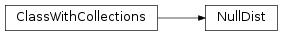# mvpa2.clfs.stats.NullDist¶class `mvpa2.clfs.stats.``NullDist`(tail='both', **kwargs)

Base class for null-hypothesis testing.

Attributes

 `descr` Description of the object if any `tail`

Methods

 `cdf`(x) Implementations return the value of the cumulative distribution function. `dists`() Implementations returns a sequence of the `dist_class` instances that were used to fit the distribution. `fit`(measure, ds) Implement to fit the distribution to the data. `p`(x[, return_tails]) Returns the p-value for values of `x`. `rcdf`(x) Implementations return the value of the reverse cumulative distribution function. `reset`()
Parameters: tail : {‘left’, ‘right’, ‘any’, ‘both’} Which tail of the distribution to report. For ‘any’ and ‘both’ it chooses the tail it belongs to based on the comparison to p=0.5. In the case of ‘any’ significance is taken like in a one-tailed test. enable_ca : None or list of str Names of the conditional attributes which should be enabled in addition to the default ones disable_ca : None or list of str Names of the conditional attributes which should be disabled descr : str Description of the instance

Attributes

 `descr` Description of the object if any `tail`

Methods

 `cdf`(x) Implementations return the value of the cumulative distribution function. `dists`() Implementations returns a sequence of the `dist_class` instances that were used to fit the distribution. `fit`(measure, ds) Implement to fit the distribution to the data. `p`(x[, return_tails]) Returns the p-value for values of `x`. `rcdf`(x) Implementations return the value of the reverse cumulative distribution function. `reset`()
`cdf`(x)

Implementations return the value of the cumulative distribution function.

`dists`()

Implementations returns a sequence of the `dist_class` instances that were used to fit the distribution.

`fit`(measure, ds)

Implement to fit the distribution to the data.

`p`(x, return_tails=False, **kwargs)

Returns the p-value for values of `x`. Returned values are determined left, right, or from any tail depending on the constructor setting.

In case a `FeaturewiseMeasure` was used to estimate the distribution the method returns an array. In that case `x` can be a scalar value or an array of a matching shape.

`rcdf`(x)

Implementations return the value of the reverse cumulative distribution function.

`tail`